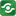Probability 1@import url(http://www.google.com/cse/api/branding.css);CSGNetwork Custom SearchCSGNetwork Snippets Calculators Top Free Apps Home Back

Right click this window and select "view source" in order to copy the source for this script.

## Calculate probability Q from z

One of the most common probability calculations is determining, given the measured z value from an experiment or set of experiments, the probability of the result being due to chance. Enter the z value in the box below, press the Return key or the Calculate button, and the probability will appear in the Q box.

Given z =

The chance probability, Q, is: or about one in